### NCERT Solutions For Class 9 Math Chapter – 12 Exercise – 12.2

NCERT Solutions For Class 9 Math Chapter – 12 Exercise – 12.2

Q1.  A park, in the shape of a quadrilateral ABCD, has DC = 900. AB = 9 m BC = 12 m , CD = 5 m and AD = 8 m , How much area does it occupy ?

Solution:-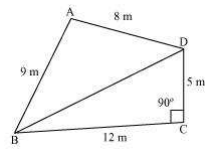BD = √(5)2 + (12)2

BD = √25 + 144 m

BD = √169 m

BD = 13 m

Area(ABCD) = Area(ABD) + Area(BCD)

Area(BCD) = ½ x 12 x 5

= 30 m2.

For triangle ABD,

S = 8+9+13/2

= 15 m

Area(ABD) = √15(15-9)(15-8)(15-13)

= √15 X 6 X 7 X 2

=

6√35 m2

= 35.5 m2.

Substituting area from (ii) and (iii) in (i) we get

Area(ABCD) = 35.5 + 30 = 65.5 m2.

Q2. Find the area of a quadrilateral ABCD in which AB = 3 cm BC = 4 cm CD = 4 cm DA = 5 cm and AC = 5 cm.

Solution:-

Area(ABCD) = Area(ABC) + Area(ACD)

For ∆ABC,

S = 3 + 4 + 5/2

S = 6 cm

Area(ABC) = √6(6-3)(6-4)(6-5) cm2

= √6x3x2x1

= 6 cm2.

In ∆ACD

S = 5+5+4/2

S = 7 cm

Area(ACD) = √7(7-5)(7-5)(7-4) cm3

= √7x2x2x3

= 2√21 cm2

= 9.17 cm2.

Substituting the values of area from (ii) and (iii) in (i) we get

Area(ABCD) = (6+9.17) cm2

= 15.17 cm2.

Q3. Radha made a picture of an aeroplane with coloured paper as shown in figure given below. Find the total area of the paper used.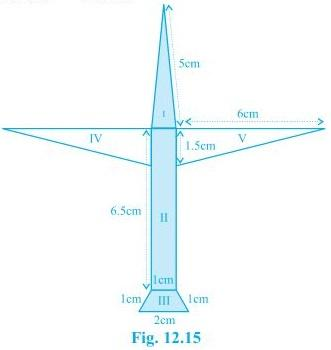Solution:-

Region 1:

S = 5 + 5 + 1 /2

S = 5.5 cm

Area(1) = √5.5(5.5-5)(5.5-5)(5.5-1)

= √5.5 x 0.5 x 0.5 x 4.5 cm2

= √6.19 cm2.

= 2.5 cm2 approx

Region II There is a rectangle.

Area(II) = 6.5 x 1 cm2 = 6.5 cm2.

Region(III) = There is a rectangle.

Parallel sides are 2 cm , 1 cm and other sides are 1 cm and 1 cm

Look the trapezium in the adjacent figure.

AL = 0.5 cm

DL = √(1)2 – (0.5)2

DL = √0.75 cm

DL = 0.9 cm

Area(III) = ½(2+1) X 0.9

= 3 X 0.45

= 1.35 cm2.

Region IV and V There are two equal right angled triangles.

For each , two perpendicular sides are 6 cm and 1.5 cm.

Area(IV and V) = 2 x ½ x 6 x 1.5 = 9 cm2.

Total area of the paper used

= Area (I + II + III + IV + V)

= (2.5 + 6.5 + 1.35 + 9.0)

= 19.35 cm2.

Hence the required area is approximately 19.4 cm3.

Q4. A triangle and a parallelogram have the same base and the same area. If the sides of the triangle are 26 cm , 28 cm and 30 cm and the parallelogram stands on the base 28 cm , find the height of the parallelogram.

Solution:-

S = 26 + 28 + 30/2

S = 42.

Area of triangle = √42(42-26)(42-28)(42-30) cm2.

= √42x16x14x12

= 336 cm2.

Area of the parallelogram = base x height

= 28 x h

From (i) we have

Substituting the values of area from (ii) and (iii) in (i) we have

28 x h = 336

H = 12 cm.

Hence height of the parallelogram = 12 cm

Q5. A rhombus shaped field has green grass for 18 cows to graze. If each side of the rhombus is 30 m and its longer diagonal is 48 m how much area of grass field will each cow be getting ?

Solution:-

DO = √(30)2 – (24)2

= √324 m

= 18 m

DB = 2 X 18 m = 36 m.

Area of the rhombus = ½ x 48 x 36

= 864 m2.

Area of grass field for each cow = 864/18 m2 = 48 m2.

Q6. An umbrella is made by stitching 10 triangular pieces of cloth of two different colours Each piece measuring 20 cm , 50 cm and 50 cm How much cloth of each colour is required for the umbrella ?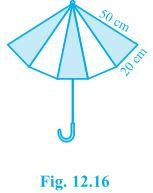Solution:-

S = 50 + 50 + 20/2

S = 60 cm.

Area of one piece = √60(60-50)(60-50)(60-20)

= 200√6 cm2.

Area of cloth of each colour = 5 x 200√6

= 1000√6 cm2.

Q7. A kite in the shape of a square with a diagonal 32 cm and an isosceles triangle base 8 cm and sides 6 cm each is to be made of three different shades as shown in figure. How much paper of each shade has been used in it?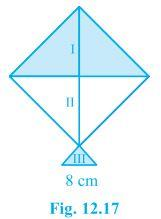Solution:-

Area of square = ½ x 32 x 32 cm2.

= 512 cm2.

Paper used for each of the shades I and II  = 512/2

= 256 cm2.

Dimensions of triangle are 8 cm , 6 cm and 6 cm.

S = 8 + 6 + 6/2

S = 10 cm

Area of shade III = √10(10-8)(10-6)(10-6) cm2

= √10x2x4x4

= 8√5 cm2.

= 17.89 cm2.

Q8.  A floral design on a floor is made up of 16 tiles which are triangular the sides of the triangle being 9 cm , 28 cm and 35 cm Find the cost of polishing the tiles at the rate of 50 per cm2.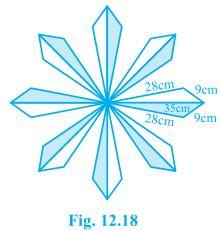Solution:-

S = 28+35+9/2

S = 72/2

S = 36 cm.

Area = √36(36-28)(36-35)(36-9)

=  √36x8x1x27

= 88.20 cm2.

Cost of polishing 16 tiles = 50/100 x 16 x 88.20

= Rs 705.60.

Q9. A field is in the shape of a trapezium whose parallel sides are 25 m and 10 m. The non – parallel sides are 14 m and 13 m. Find the area of the field.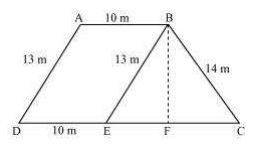Solution:-

S = 14 + 15 + 13/2

S = 21 m.

Area of triangle BCE = √21(21-14)(21-15)(21-13)

= √21 x 7 x 6 x 8 m2

= 84 m2

Let height of the triangle = h m

84 = ½ x 15 x h

H = 84×2/15 m

H = 56/5 m

Area of the field = ½(10+25) x 56/5 m2.

= ½ x 35 x 56/5 m2

= 196 m2 .